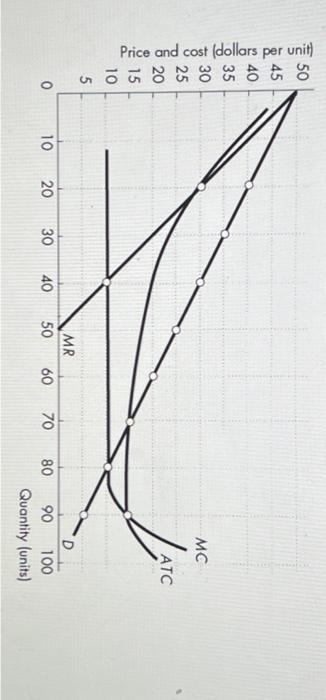Home / Expert Answers / Economics / this-graph-shows-a-natural-monopoly-1-if-the-firm-is-left-unregulated-what-would-be-the-price-and-pa370

# (Solved): This graph shows a natural monopoly. 1. If the firm is left unregulated, what would be the price and ...

This graph shows a natural monopoly.

1. If the firm is left unregulated, what would be the price and quantity?

a. What would be the amount of the firms economic profit or loss?

b. What is the amount of deadweight loss?

2. If the firm was regulated with an average cost pricing rule, what would be the price and quantity?

a. What would be the amount of the firms economic profit or loss?

b. What is the amount of the deadweight loss?

3. By how much does the consumer surplus change when the firm changes from being unregulated to regulated with a marginal cost pricing rule?Price and cost (dollars per unit)

We have an Answer from Expert